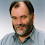## Tuesday, May 8, 2012

### A fundamental limitation of hybridization networks? (2)

This is a follow-up to an earlier post, which showed an example of two phylogenetic trees and three rooted phylogenetic networks. You can see them again in the figure below.

Each of the networks N1, N2 and N3 displays the two trees T1 and T2 (and no other trees). Thus, it is impossible to decide which of the three networks is correct. The question was asked whether this is a fundamental limitation of rooted phylogenetic networks (a.k.a. hybridization networks).

In my opinion, the answer is "no".

Let's first draw the networks such that each reticulation is an instantaneous event between two coexisting taxa. To do so, networks N2 and N3 need an additional taxon x, which could be an extinct taxon or just a taxon that has not been sampled.

I've specified a length for each edge of each network and have given corresponding edge lengths to the trees. The values of the edge lengths in the networks have been chosen rather arbitrarily, and are not important for the discussion below.

What is important is that, when you take the edge lengths into account, it is easy to decide which of the three networks should be chosen. N1 should be chosen if the roots of T1 and T2 have the same age, N2 should be chosen if the root of T1 is older and N3 if the root of T2 is older. The reason is the following. In network N1, the roots of T1 and T2 both coincide with the root of the network. This contrasts with network N2, where the root of T2 is a proper descendant of  the root of T1 and with network N3, in which the root of T1 is a proper descendant of the root of T2.

We can conclude that the above example shows an important challenge but not a fundamental limitation of rooted phylogenetic networks. When taking edge lengths into account, it is indeed possible to uniquely reconstruct the network (at least in this case).

1.Leo's suggestion is a very interesting one. Branch lengths have rarely been considered in algorithms for constructing hybridization networks, although they have often played an important role in phylogenetic trees. Thus, Leo's approach to inferring the optimal network based on relative branch lengths highlights the need to use ALL of the information from the component trees when constructing a hybridization network — tree topology may be necessary but is not sufficient.

Importantly, what Leo's analysis requires from biologists is that the edge lengths of their trees need to represent time, at least in terms of their relative (but not necessarily absolute) lengths. That is, we need to know the relative time order of the branching points in the trees. If different edge lengths represent, for example, differences in the number of inferred character-state changes resulting from variation in evolutionary rate, then the logic may not hold. Unfortunately, this is what they DO represent in most phylogenetic trees.

It thus seems to me that the "fundamental limitation" is going to be the ability of biologists to produce so-called 'time trees' when they wish to build networks explicitly representing evolutionary history. This has not been a requirement when building the trees themselves, where topology has frequently been considered to be quite sufficient.

2.That is an interesting point, David. Indeed, if the evolutionary rate varies from gene to gene and from branch to branch, then the above reasoning does not hold. Nevertheless, it might still be possible to use edge-lengths to uniquely reconstruct the network, as long as the evolutionary rate doesn't change too much. There might also be other information that one might be able to take into account.

So the main point is, like you said, that tree topology is important but that additional information is necessary to reconstruct networks.